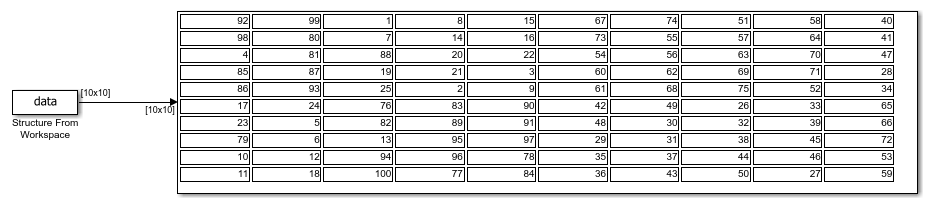# Read 2-D Signals in Structure Format From Workspace

This example shows how to read a 2-D structure from the MATLAB workspace. When you open the model, the following code is executed by a `PreLoadFcn` callback:

```t1 = 0.2 * [0:49]'; m = magic(10); M = repmat(m,[1 1 length(t1)]); data.time=t1; data.signals.values = M; data.signals.dimensions=[10 10]; ```

This code creates 10-by-10 matrix (2-D signal) by using the `magic` function, and then creates a 3-D matrix by adding a time vector. The time vector must be a column vector. The `signals.values` field is a 3-D matrix where the third dimension corresponds to time. The `signals.dimensions` field is a two-element vector. The first element is the number of rows and the second element is the number of columns in the `signals.values` field.

When you run the model, the From Workspace block reads the structure `data` from the workspace.